Courses

# Chapter Notes - Practical Geometry Class 7 Notes | EduRev

## Class 7 : Chapter Notes - Practical Geometry Class 7 Notes | EduRev

The document Chapter Notes - Practical Geometry Class 7 Notes | EduRev is a part of the Class 7 Course Mathematics (Maths) Class 7.
All you need of Class 7 at this link: Class 7

10. Practical Geometry

Construction of Triangles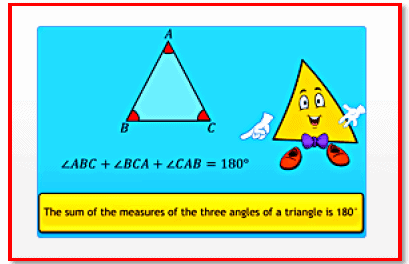The sum of the measures of the three angles .

1) The sum of the measures of the three angles of a triangle is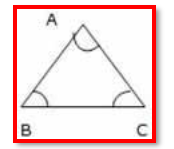2) The sum of the lengths of any two sides of a triangle is always greater than the length of the third side.

AB + BC > CA; BC + CA > AB and CA + AB > BC

3) The difference between the lengths of any two sides of a triangle is always less than the length of the third side.

AB - BC < CA; BC - CA < AB and CA - AB < BC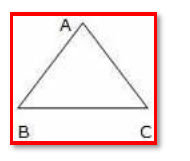Page 39

4) The measure of an exterior angle is equal to the sum of the two remote or opposite interior angles.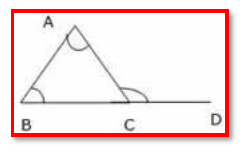Practical Geometry - Construction of Triangles

The properties of triangles are as follows:

To construct a triangle, we should know any one of the following:

Length of the three sides
Two sides and the included angle
Two angles and the included side
Length of the hypotenuse and one side in case of a right-angled triangle.

Construction of Parallel Lines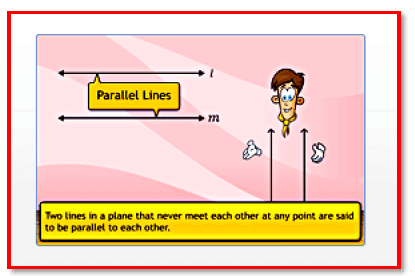Two lines in a plane that never meet each other at any point are said to be parallel to each other.

Page 40

Two lines in a plane that never meet each other at any point are said to be parallel to each other. Any line intersecting a pair of parallel lines is called a transversal.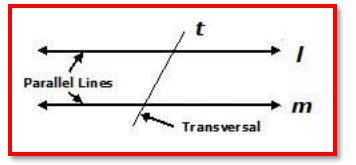Properties of angles formed by parallel lines and transversal:

1. All pairs of alternate interior angles formed by parallel lines and a transversal are equal.
2. All pairs of corresponding angles formed by parallel lines and a transversal are equal.
3. All pairs of alternate exterior angles formed by parallel lines and a transversal are equal.
4. The interior angles formed on the same side of the transversal are supplementary (the sum of their measures is 1800).

Steps to construct parallel lines using the alternate interior angle property:

1. Draw a line, l.
2. Mark point A outside line l.
3. Mark point B on line l.
4. Draw line joining points A and B.
5. Draw an arc with B as the centre, such that it intersects lines l and n at points D and E, respectively.
6. Draw another arc with the same radius and A as the centre, such that it intersects line n at F.
7. Ensure that the arc drawn from A cuts line n between A and B.
8. Measure distance DE with the help of the compass.
9. Draw another arc with F as the centre and radius equal to DE.
10. Mark the point of intersection of this arc and the previous arc as G.
11. Draw line m passing through A and G.
12. Lines l and m are parallel.

Page 41

Verification of the construction:

If the pair of alternate interior angles ∠ABC and ∠BAG are equal in measure, then line l //line m. Hence, the construction is verified.

Steps to construct parallel lines using the corresponding angle property:

1. Draw line l and point P outside it.
2. Mark point Q on line l.
3. Draw line joining point P and point Q.
4. Draw an arc with Q as the centre, such that it intersects line l at R and line n at S.
5. Draw another arc with the same radius and P as the centre, such that it intersects line n at X.
6. Ensure that the arc drawn from P cuts line n outside QP.
7. Draw another arc withX as the centre and distance RS as the radius, such that it intersects the previous arc at Y.
8. Draw line m passing through points P and Y.
9. Lines l and m are parallel.

Verification of the construction:
If the pair of corresponding angles ∠PQR and ∠XPY are equal in measure, then line l II line m.

Offer running on EduRev: Apply code STAYHOME200 to get INR 200 off on our premium plan EduRev Infinity!

## Mathematics (Maths) Class 7

161 videos|237 docs|45 tests

,

,

,

,

,

,

,

,

,

,

,

,

,

,

,

,

,

,

,

,

,

;